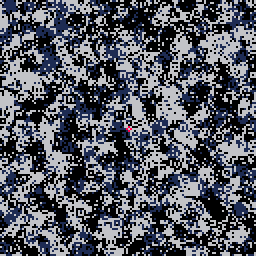My second entry for TweetTweetJam 5 ! The rule being that the final game code must be 560 characters or less.

In Make it Pink!, you have to color all the screen in pink by moving the initial pink few pixels. You can keep X pressed to get bigger, and C to play again or reboot.#### Here's the code (556 characters)

```h={1,3,4}i={5,6,15}r=rnd l=0::b::j=h[flr(r(3)+1)]k=i[flr(r(3)+1)]p={k,k,j,k,0,k,k,0,j,k,0,j}for i=0,15do pal(i,p[i])end
for x=0,127do for y=0,127do pset(x,y,r()*15)end end
x,y,d,e,o=64,64,0,0,0::_:: d+=1
if o==0and d>24then d=0e+=1
z=btn()x+=(z\2%2-z%2)/300y+=(z\8%2-z\4%2)/300pal()
if(btn(5))fillp(▒)circfill(x,y,7,8)fillp()
circ(x,y,1,8)p,q=r(128),r(128)circ(p,q,flr(r(3)),pget(p,q))
if e>999 then
e=0o=1
for a=0,127do for b=0,127do
if(pget(a,b)!=8)o=0goto w
end end::w::
end end
if o==1then o=2
?flr(t()-l).."s!",58,50,6
end if(btn(4))l=t()goto b
goto _
```
 Status Prototype Platforms HTML5 Rating Rated 4.7 out of 5 stars(3 total ratings) Author Robin Six Made with PICO-8 Tags PICO-8, tweettweetjam